Chemistry 2e

# Exercises

Chemistry 2eExercises

### 20.1Hydrocarbons

1.

Write the chemical formula and Lewis structure of the following, each of which contains five carbon atoms:

(a) an alkane

(b) an alkene

(c) an alkyne

2.

What is the difference between the hybridization of carbon atoms’ valence orbitals in saturated and unsaturated hydrocarbons?

3.

On a microscopic level, how does the reaction of bromine with a saturated hydrocarbon differ from its reaction with an unsaturated hydrocarbon? How are they similar?

4.

On a microscopic level, how does the reaction of bromine with an alkene differ from its reaction with an alkyne? How are they similar?

5.

Explain why unbranched alkenes can form geometric isomers while unbranched alkanes cannot. Does this explanation involve the macroscopic domain or the microscopic domain?

6.

Explain why these two molecules are not isomers: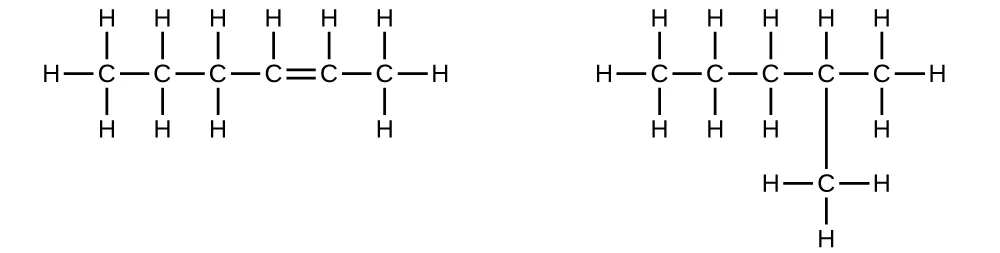7.

Explain why these two molecules are not isomers: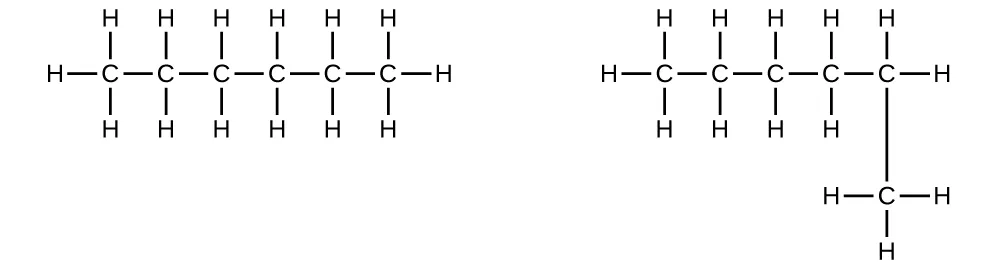8.

How does the carbon-atom hybridization change when polyethylene is prepared from ethylene?

9.

Write the Lewis structure and molecular formula for each of the following hydrocarbons:

(a) hexane

(b) 3-methylpentane

(c) cis-3-hexene

(d) 4-methyl-1-pentene

(e) 3-hexyne

(f) 4-methyl-2-pentyne

10.

Write the chemical formula, condensed formula, and Lewis structure for each of the following hydrocarbons:

(a) heptane

(b) 3-methylhexane

(c) trans-3-heptene

(d) 4-methyl-1-hexene

(e) 2-heptyne

(f) 3,4-dimethyl-1-pentyne

11.

Give the complete IUPAC name for each of the following compounds:

(a) CH3CH2CBr2CH3

(b) (CH3)3CCl

(c)(d) $CH3CH2C≡CH CH3CH2C≡CHCH3CH2C≡CH CH3CH2C≡CH$

(e)(f)(g) $(CH3)2CHCH2CH=CH2(CH3)2CHCH2CH=CH2$

12.

Give the complete IUPAC name for each of the following compounds:

(a) (CH3)2CHF

(b) CH3CHClCHClCH3

(c)(d) $CH3CH2CH=CHCH3CH3CH2CH=CHCH3$

(e)(f) $(CH3)3CCH2C≡CH(CH3)3CCH2C≡CH$

13.

Butane is used as a fuel in disposable lighters. Write the Lewis structure for each isomer of butane.

14.

Write Lewis structures and name the five structural isomers of hexane.

15.

Write Lewis structures for the cis–trans isomers of $CH3CH=CHCl.CH3CH=CHCl.$

16.

Write structures for the three isomers of the aromatic hydrocarbon xylene, C6H4(CH3)2.

17.

Isooctane is the common name of the isomer of C8H18 used as the standard of 100 for the gasoline octane rating:(a) What is the IUPAC name for the compound?

(b) Name the other isomers that contain a five-carbon chain with three methyl substituents.

18.

Write Lewis structures and IUPAC names for the alkyne isomers of C4H6.

19.

Write Lewis structures and IUPAC names for all isomers of C4H9Cl.

20.

Name and write the structures of all isomers of the propyl and butyl alkyl groups.

21.

Write the structures for all the isomers of the –C5H11 alkyl group.

22.

Write Lewis structures and describe the molecular geometry at each carbon atom in the following compounds:

(a) cis-3-hexene

(b) cis-1-chloro-2-bromoethene

(c) 2-pentyne

(d) trans-6-ethyl-7-methyl-2-octene

23.

Benzene is one of the compounds used as an octane enhancer in unleaded gasoline. It is manufactured by the catalytic conversion of acetylene to benzene:
$3C2H2⟶C6H63C2H2⟶C6H6$

Draw Lewis structures for these compounds, with resonance structures as appropriate, and determine the hybridization of the carbon atoms in each.

24.

Teflon is prepared by the polymerization of tetrafluoroethylene. Write the equation that describes the polymerization using Lewis symbols.

25.

Write two complete, balanced equations for each of the following reactions, one using condensed formulas and one using Lewis structures.

(a) 1 mol of 1-butyne reacts with 2 mol of iodine.

(b) Pentane is burned in air.

26.

Write two complete, balanced equations for each of the following reactions, one using condensed formulas and one using Lewis structures.

(a) 2-butene reacts with chlorine.

(b) benzene burns in air.

27.

What mass of 2-bromopropane could be prepared from 25.5 g of propene? Assume a 100% yield of product.

28.

Acetylene is a very weak acid; however, it will react with moist silver(I) oxide and form water and a compound composed of silver and carbon. Addition of a solution of HCl to a 0.2352-g sample of the compound of silver and carbon produced acetylene and 0.2822 g of AgCl.

(a) What is the empirical formula of the compound of silver and carbon?

(b) The production of acetylene on addition of HCl to the compound of silver and carbon suggests that the carbon is present as the acetylide ion, $C22−C22−$. Write the formula of the compound showing the acetylide ion.

29.

Ethylene can be produced by the pyrolysis of ethane:
$C2H6⟶C2H4+H2C2H6⟶C2H4+H2$

How many kilograms of ethylene is produced by the pyrolysis of 1.000 $××$ 103 kg of ethane, assuming a 100.0% yield?

### 20.2Alcohols and Ethers

30.

Why do the compounds hexane, hexanol, and hexene have such similar names?

31.

Write condensed formulas and provide IUPAC names for the following compounds:

(a) ethyl alcohol (in beverages)

(b) methyl alcohol (used as a solvent, for example, in shellac)

(c) ethylene glycol (antifreeze)

(d) isopropyl alcohol (used in rubbing alcohol)

(e) glycerine

32.

Give the complete IUPAC name for each of the following compounds:

(a)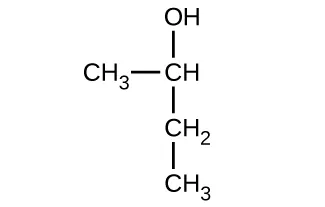(b)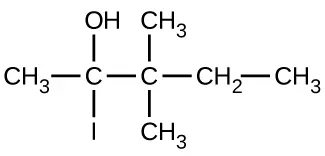(c)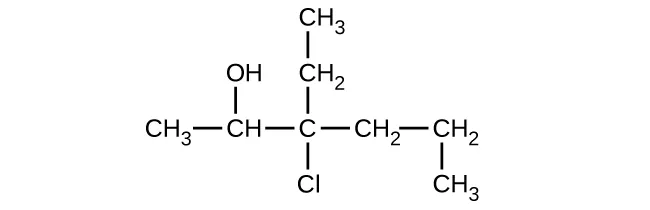33.

Give the complete IUPAC name and the common name for each of the following compounds:

(a)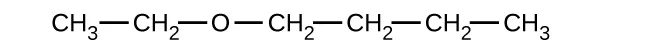(b)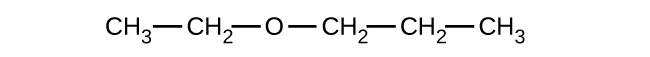(c)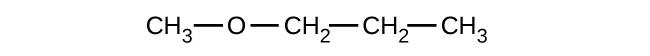34.

Write the condensed structures of both isomers with the formula C2H6O. Label the functional group of each isomer.

35.

Write the condensed structures of all isomers with the formula C2H6O2. Label the functional group (or groups) of each isomer.

36.

Draw the condensed formulas for each of the following compounds:

(a) dipropyl ether

(b) 2,2-dimethyl-3-hexanol

(c) 2-ethoxybutane

37.

MTBE, Methyl tert-butyl ether, CH3OC(CH3)3, is used as an oxygen source in oxygenated gasolines. MTBE is manufactured by reacting 2-methylpropene with methanol.

(a) Using Lewis structures, write the chemical equation representing the reaction.

(b) What volume of methanol, density 0.7915 g/mL, is required to produce exactly 1000 kg of MTBE, assuming a 100% yield?

38.

Write two complete balanced equations for each of the following reactions, one using condensed formulas and one using Lewis structures.

(a) propanol is converted to dipropyl ether

(b) propene is treated with water in dilute acid.

39.

Write two complete balanced equations for each of the following reactions, one using condensed formulas and one using Lewis structures.

(a) 2-butene is treated with water in dilute acid

(b) ethanol is dehydrated to yield ethene

### 20.3Aldehydes, Ketones, Carboxylic Acids, and Esters

40.

Order the following molecules from least to most oxidized, based on the marked carbon atom: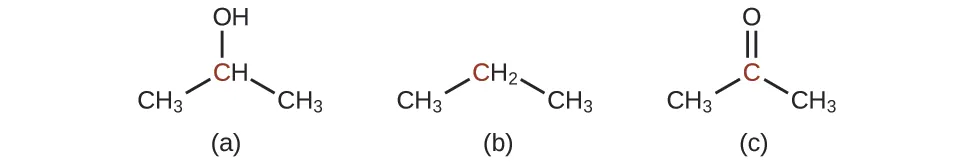41.

Predict the products of oxidizing the molecules shown in this problem. In each case, identify the product that will result from the minimal increase in oxidation state for the highlighted carbon atom:

(a)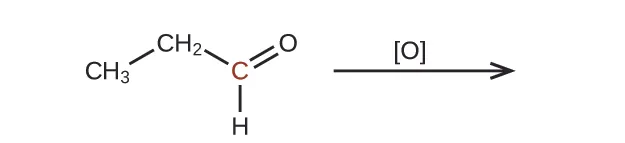(b)(c)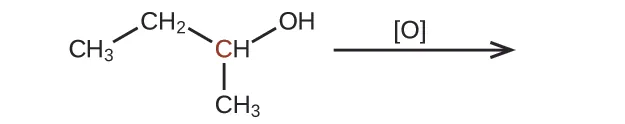42.

Predict the products of reducing the following molecules. In each case, identify the product that will result from the minimal decrease in oxidation state for the highlighted carbon atom:

(a)(b)(c)43.

Explain why it is not possible to prepare a ketone that contains only two carbon atoms.

44.

How does hybridization of the substituted carbon atom change when an alcohol is converted into an aldehyde? An aldehyde to a carboxylic acid?

45.

Fatty acids are carboxylic acids that have long hydrocarbon chains attached to a carboxylate group. How does a saturated fatty acid differ from an unsaturated fatty acid? How are they similar?

46.

Write a condensed structural formula, such as CH3CH3, and describe the molecular geometry at each carbon atom.

(a) propene

(b) 1-butanol

(c) ethyl propyl ether

(d) cis-4-bromo-2-heptene

(e) 2,2,3-trimethylhexane

(f) formaldehyde

47.

Write a condensed structural formula, such as CH3CH3, and describe the molecular geometry at each carbon atom.

(a) 2-propanol

(b) acetone

(c) dimethyl ether

(d) acetic acid

(e) 3-methyl-1-hexene

48.

The foul odor of rancid butter is caused by butyric acid, CH3CH2CH2CO2H.

(a) Draw the Lewis structure and determine the oxidation number and hybridization for each carbon atom in the molecule.

(b) The esters formed from butyric acid are pleasant-smelling compounds found in fruits and used in perfumes. Draw the Lewis structure for the ester formed from the reaction of butyric acid with 2-propanol.

49.

Write the two-resonance structures for the acetate ion.

50.

Write two complete, balanced equations for each of the following reactions, one using condensed formulas and one using Lewis structures:

(a) ethanol reacts with propionic acid

(b) benzoic acid, C6H5CO2H, is added to a solution of sodium hydroxide

51.

Write two complete balanced equations for each of the following reactions, one using condensed formulas and one using Lewis structures.

(a) 1-butanol reacts with acetic acid

(b) propionic acid is poured onto solid calcium carbonate

52.

Yields in organic reactions are sometimes low. What is the percent yield of a process that produces 13.0 g of ethyl acetate from 10.0 g of CH3CO2H?

53.

Alcohols A, B, and C all have the composition C4H10O. Molecules of alcohol A contain a branched carbon chain and can be oxidized to an aldehyde; molecules of alcohol B contain a linear carbon chain and can be oxidized to a ketone; and molecules of alcohol C can be oxidized to neither an aldehyde nor a ketone. Write the Lewis structures of these molecules.

### 20.4Amines and Amides

54.

Write the Lewis structures of both isomers with the formula C2H7N.

55.

What is the molecular structure about the nitrogen atom in trimethyl amine and in the trimethyl ammonium ion, (CH3)3NH+? What is the hybridization of the nitrogen atom in trimethyl amine and in the trimethyl ammonium ion?

56.

Write the two resonance structures for the pyridinium ion, C5H5NH+.

57.

Draw Lewis structures for pyridine and its conjugate acid, the pyridinium ion, C5H5NH+. What are the hybridizations, electron domain geometries, and molecular geometries about the nitrogen atoms in pyridine and in the pyridinium ion?

58.

Write the Lewis structures of all isomers with the formula C3H7ON that contain an amide linkage.

59.

Write two complete balanced equations for the following reaction, one using condensed formulas and one using Lewis structures.

Methyl amine is added to a solution of HCl.

60.

Write two complete, balanced equations for each of the following reactions, one using condensed formulas and one using Lewis structures.

Ethylammonium chloride is added to a solution of sodium hydroxide.

61.

Identify any carbon atoms that change hybridization and the change in hybridization during the reactions in Exercise 20.26.

62.

Identify any carbon atoms that change hybridization and the change in hybridization during the reactions in Exercise 20.39.

63.

Identify any carbon atoms that change hybridization and the change in hybridization during the reactions in Exercise 20.51.

Order a print copy

As an Amazon Associate we earn from qualifying purchases.